# Moments (Part - 2) - Forces and Moments Civil Engineering (CE) Notes | EduRev

## Civil Engineering (CE) : Moments (Part - 2) - Forces and Moments Civil Engineering (CE) Notes | EduRev

The document Moments (Part - 2) - Forces and Moments Civil Engineering (CE) Notes | EduRev is a part of the Civil Engineering (CE) Course Introduction to Dynamics and Vibrations- Notes, Videos, MCQs.
All you need of Civil Engineering (CE) at this link: Civil Engineering (CE)

2.2.5 A few tips on calculating moments

The safest way to calculate the moment of a force is to slog through the MA = (r− rA) × F formula, as described at the start of this section. As long as you can write down position vectors and force vectors correctly, and can do a cross product, it is totally fool-proof.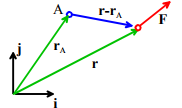But if you have a good physical feel for forces and their effects you might like to make use of the following short cuts.

1. The direction of a moment is always perpendicular to both (r - rA) and F. For 2D problems (r - rA) and F lie in the same plane, so the direction of the moment must be perpendicular to this plane.

Thus, a set of 2D forces in the {i,j} plane can only exert moments in the ±k direction – this makes calculating moments in 2D problems rather simple; we just have to figure out whether the sign of a moment is positive or negative.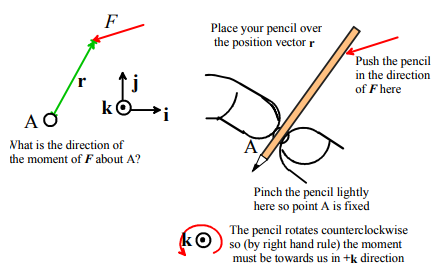You can do a quick experiment to see whether the direction is +k or –k. Suppose you want to find the direction of the moment caused by F in the picture above about the point A. To do so,

(i) Place your pencil on the page so that it lies on the line connecting A to the force.
(ii) Pinch the pencil lightly at A so it can rotate about A, but A remains fixed.
(iii) Push on the pencil in the direction of the force at B. If the pencil rotates counterclockwise, the direction of the moment of F about A is out of the picture (usually +k). If it rotates clockwise, the direction of the moment is into the picture (–k). If it doesn’t rotate, you’re either holding the pencil in a death grip at A (then the experiment won’t work) or else the force must be acting along the pencil – in this case the moment is zero.

In practice you will soon find that you can very quickly tell the direction of a moment (in 2D, anyway) just by looking at the picture, but the experiment might help until you develop this intuition.

2. The magnitude of a moment about some point is equal to the perpendicular distance from that point to the line of action of the force, multiplied by the magnitude of the force.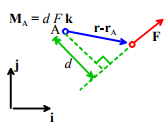Again, this trick is most helpful in 2D. Its use is best illustrated by example. Let’s work through the simple 2D example problems again, but now use the short-cut.

Example 1: The beam shown below is uniform and has weight W. Calculate the moment exerted by the gravitational force about points A and B.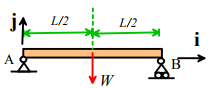The perpendicular distance from a vertical line through the CG to A is L/2. The pencil trick shows that W exerts a clockwise moment about A. Therefore

MA = −( LW/ 2)k

Similarly, the perpendicular distance to B is L/2, and W exerts a counterclockwise moment about B. Therefore

MB = (LW/2)k

Example 2. Member AB of a roof-truss is subjected to a vertical gravitational force W and a horizontal wind load P. Calculate the moment of the resultant force about B.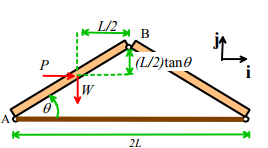The perpendicular distance from the line of action of W to B is L/2. W exerts a counterclockwise moment about B. Therefore W exerts a moment MB = (L/2)Wk

The perpendicular distance from the line of action of P to B is (L/ 2) tan θ . P also exerts a counterclockwise moment about B. Therefore

MB = (L tan θ/ 2) Pk

The total moment is

MB = ( L/ 2){W+ P tanθ}k

Example 3: It is traditional in elementary statics courses to solve lots of problems involving ladders (oh boy! Aren’t you glad you signed up for engineering?) . The picture below shows a ladder of length L and weight W resting on the top of a frictionless wall. Forces acting on the ladder are shown as well. Calculate the moments about point A of the reaction force at B (which acts perpendicular to the ladder) and the weight force at C (which acts at the center of gravity, half-way up the ladder).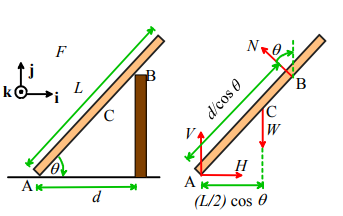The perpendicular distance from point A to the line along which N acts is d / cosθ. The pencil experiment (or inspection) shows that the direction of the moment of N about A is in the +k direction. Therefore the trick (perpendicular distance times force) gives

MA = (d / cosθ )N k (for the force acting at B)

The perpendicular distance from point A to the line along which W is acting is (L/ 2) cosθ .  The direction of the moment is –k.  Therefore

MA =−(L / 2) cosθWk (for the weight force)

Let’s compare these with the answer we get using MA = (r - rA)× F. We can take the origin to be at A to make things simple.  Then, for the force at B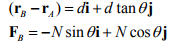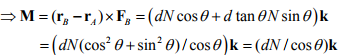giving the same answer as before, but with a whole lot more effort!

Similarly, for the weight force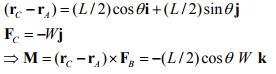3. The moment exerted by a force is unchanged if the force is moved in a direction parallel to the direction of the force.

This is rather obvious in light of trick (2), but it’s worth stating anyway.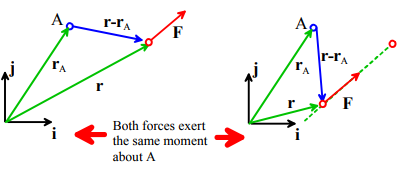4. The component of moment exerted by a force about an axis through a point can be calculated by (i) finding the two force components perpendicular to the axis; then (ii) multiplying each force component by its perpendicular distance from the axis; and (iii) adding the contributions of each force component following the right-hand screw convention.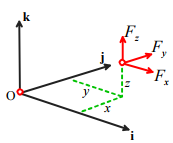The wording of this one probably loses you, so let’s start by trying to explain what this means.

First, let’s review what we mean by the component of a moment about some axis. The formula for the moment of a force about the origin is

Mo = {yFz− zFy}i + {zFx − xFz} j + {xFy − yFz} k

This has three components - Mx = {yFz - zFy} about the i axis, My = {zFx = − xFz} about the j axis, and Mz = {xFy - yFx} about the k axis.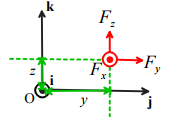The trick gives you a quick way to calculate one of the components. For example, let’s try to find the i component of the moment about the origin exerted by the force shown in the picture.

The rule says
(i) Identify the force components perpendicular to the i axis – that’s Fz and Fy in this case;
(ii) Multiply each force component by its perpendicular distance from the axis. Drawing a view down the i axis is helpful. From the picture, we can see that Fz is a distance y from the axis, and Fy is a distance z from the axis. The two contributions we need are thus yFz and zFy.
(iii) Add the two contributions according to the right hand screw rule. We know that each force component exerts a moment ±i - we have to figure out which one is +i and which is –i. We can do the pencil experiment to figure this out – the answer is that Fz exerts a moment along +i, while Fy causes a moment along –i. So finally

Mx = − {yFz - zFy}.

Example: The structure shown is subjected to a vertical force V and horizontal force H acting at E. Calculate the k component of moment exerted about point A by the resultant force.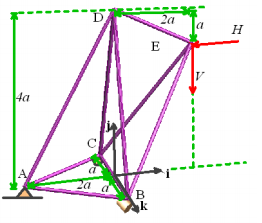Our trick gives the answer immediately. First, draw a picture looking down the k axis

Clearly, the force H exerts a k component of moment 3aHk , while the force V exerts a k component of moment −4aV k . The total k component of moment is

MAz = a(3H− 4V)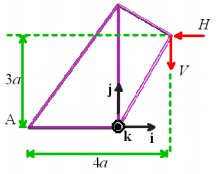This trick clearly can save a great deal of time. But to make use of it, you need excellent 3D visualization skills.

Offer running on EduRev: Apply code STAYHOME200 to get INR 200 off on our premium plan EduRev Infinity!

## Introduction to Dynamics and Vibrations- Notes, Videos, MCQs

20 videos|53 docs

,

,

,

,

,

,

,

,

,

,

,

,

,

,

,

,

,

,

,

,

,

;